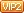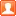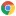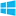# 比特彗星把smb局域网nas的文件保种，下载任务修改xml实现跳过校验直接开始上传批量做种办法成长值: 1295

[LV.Master]伴坛终老发表于 2023/6/24 16:26 | 显示全部楼层 |阅读模式 |Google Chrome 114.0.0.0|Windows 10

 比特彗星把smb局域网nas的文件保种，下载任务修改xml实现跳过校验直接开始上传批量做种办法 几百个种子的话，一个一个校验挺麻烦的，通过研究，发现是torrents目录下xml文件，BitField字段保存完成信息，用字符f代替，留空代表进度为0 ^.*(.*)\$复制代码 替换成 ffffffffffffffffffffffffffffffffffffffffffffffffffffffffffffffffffffffffffffffffffffffffffffffffffffffffffffffffffffffffffffffffffffffffffffffffffffffffffffffffffffffffffffffffffffffffffffffffffffffffffffffffffffffffffffffffffffffffffffffffffffffffffffffffffffffffffffffffffffffffffffffffffffffffffffffffffffffffffffffffffffffffffffffffffffffffffffffffffffffffffffffffffffffffffffffffffffffffffffffffffffffffffffffffffffffffffffffffffffffffffffffffffffffffffffffffffffffffffffffffffffffffffffffffffffffffffffffffffffffffffffffffffffffffffffffffffffffffffffffffffffffffffffffffffffffffffffffffffffffffffffffffffffffffffffffffffffffffffffffffffffffffffffffffffffffffffffffffffffffffffffffffffffffffffffffffffffffffffffffffffffffffffffffffffffffffffffffffffffffffffffffffffffffffffffffffffffffffffffffffffffffffffffffffffffffffffffffffffffffffffffffffffffffffffffffffffffffffffffffffffffffffffffffffffffffffffffffffffffffffffffffffffffffffffffffffffffffffffffffffffffffffffffffffffffffffffffffffffffffff复制代码 此时打开比特彗星开始任务，进度条直接变成100%

### 评分

 本版积分规则 回帖后跳转到最后一页

GMT+8, 2023/12/4 05:37 , Processed in 0.109899 second(s), 23 queries , MemCache On.